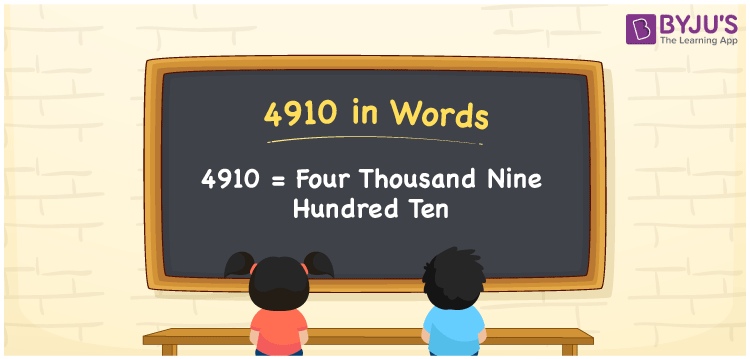# 4910 in words

4910 in wordsis written as Four Thousand Nine Hundred and Ten. 4910 represents the count or value. The article on Counting Numbers can give you an idea about count or counting. The number 4910 is used in expressions that relate to money, distance, length and others. Let us consider an example for 4910. ”The altitude of this mountain is Four Thousand Nine Hundred and Ten meters.”

 4910 in words Four Thousand Nine Hundred and Ten Four Thousand Nine Hundred and Ten in Numbers 4910

## 4910 in English Words## How to Write 4910 in Words?

We can convert 4910 to words using a place value chart. The number 4910 has 4 digits, so let’s make a chart that shows the place value up to 4 digits.

 Thousands Hundreds Tens Ones 4 9 1 0

Thus, we can write the expanded form as:

4 × Thousand + 9 × Hundred + 1 × Ten + 0 × One

= 4 × 1000 + 9 × 100 + 1 × 10 + 0 × 1

= 4910

= Four Thousand Nine Hundred and Ten.

4910 is the natural number that is succeeded by 4909 and preceded by 4911.

4910 in words – Four Thousand Nine Hundred and Ten.

Is 4910 an odd number? – No.

Is 4910 an even number? – Yes.

Is 4910 a perfect square number? – No.

Is 4910 a perfect cube number? – No.

Is 4910 a prime number? – No.

Is 4910 a composite number? – Yes.

## Solved Example

1. Write the number 4910 in expanded form

Solution: 4 × 1000 + 9 × 100 + 1 × 10 + 0 × 1

We can write 4910 = 4000 + 900 + 10 + 0

= 4 × 1000 + 9 × 100 + 1 × 10 + 0 × 1

## Frequently Asked Questions on 4910 in words

Q1

### How to write 4910 in words?

4910 in words is written as Four Thousand Nine Hundred and Ten.
Q2

### Is 4910 a perfect square number?

No. 4910 is not a perfect square number.
Q3

### Is 4910 a prime number?

No. 4910 is not a prime number.